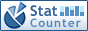Home PC Games Linux Windows Database Network Programming Server Mobile
 Home \ Programming \ Python implementation Bursa transition model - C ++ Learning Notes: references (Programming) - Memcached distributed caching (Server) - C / C ++ language usage summary of const (Programming) - Linux group account deletion, and other related operations (Linux) - Python context managers (Programming) - Adding SSH to Github (Linux) - MongoDB 2.6 deployment replica set + partitions (Database) - Multipath configuration under Linux (Linux) - Linux user status query, and to unlock locked user (Linux) - Docker use Dockerfile created since the launch of the service support SSH container mirror (Server) - Ubuntu 15.10 installation and deployment Swift development environment (Linux) - JavaScript closures and the scope chain (Programming) - Ubuntu PPA install SMPlayer 14.9 (Linux) - Bash common several configuration files (Linux) - OpenSSL Introduction and compilation steps on Windows, Linux, Mac systems (Linux) - CentOS 6.4 dial-up Raiders (Linux) - Quick paging ROW_NUMBER conducted (Database) - Vim custom color (Linux) - CentOS installation of the ftp (Linux) - Why HBase need to build SQL engine layer (Database) Python implementation Bursa transition model Add Date : 2018-11-21 The core issue 1. Because it is the same accuracy and mutually independent observations, final weight matrix should be used for the matrix P (3m * 3m), m is the number of known points. p = np.eye (n) # Unit weight matrix 3n * 3n 2. The program implements all known points of XYZ coordinate read into the corresponding columns of the matrix, the coefficient matrix B also need this operation (V = BX-L). When numpy process can be expressed as: for i in range (vector_count):         matrix_source.append (vector3d_list_source [i] .X)         matrix_source.append (vector3d_list_source [i] .Y)         matrix_source.append (vector3d_list_source [i] .Z)         matrix_dest.append (vector3d_list_dest [i] .X)         matrix_dest.append (vector3d_list_dest [i] .Y)         matrix_dest.append (vector3d_list_dest [i] .Z)         matrix_B.append ([1, 0, 0, 0, -vector3d_list_source [i] .Z, vector3d_list_source [i] .Y, vector3d_list_source [i] .X])         matrix_B.append ([0, 1, 0, vector3d_list_source [i] .Z, 0, -vector3d_list_source [i] .X, vector3d_list_source [i] .Y])         matrix_B.append ([0, 0, 1, -vector3d_list_source [i] .Y, vector3d_list_source [i] .X, 0, vector3d_list_source [i] .Z])     matrix_source = np.array (matrix_source) .reshape (1, -1) .T     matrix_dest = np.array (matrix_dest) .reshape (1, -1) .T     matrix_B = np.array (matrix_B)     L = matrix_dest - matrix_source 3. The value of the parameter matrix (column matrix) X = np.dot (np.linalg.inv (np.dot (matrix_B.T, matrix_B)), np.dot (matrix_B.T, L)) 4. The error equation V = np.dot (matrix_B, X) - L # error equation 5. Accuracy Assessment standard_deviation = math.sqrt (np.dot (np.dot (V.T, p), V) / r) # conversion error More:
 CopyRight 2002-2022 newfreesoft.com, All Rights Reserved.